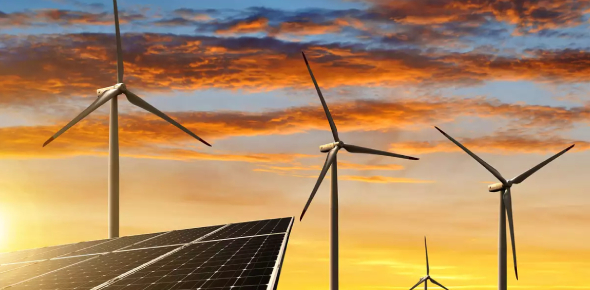# Quiz: Basic Practice Questions On Energy!

10 Questions | Total Attempts: 150SettingsEnergy exists in different forms and this can either be in the form of heat, light or Motion. There is much that we got to cover when it comes to energy in grade 6 to 8 and the test below is designed to help you see just how much you recall when it comes to energy and the different forms it takes. Take it up and see how high your score is.

• 1.
1.  What is energy efficiency?
• A.

Using as little electricity as you possibly can

• B.

Using less energy to do the same (or more) work

• C.

Doing less work to save electricity

• D.

Giving up modern comforts

• 2.
2.  Which type of light bulb is the MOST efficient?
• A.

Compact Fluorescent Light Bulb (CFL)

• B.

Incandescent Light Bulb

• C.

Light Emitting Diode Light Bulb (LED)

• D.

• 3.
3.  Up to _______ of energy used in schools is WASTED.
• A.

50%

• B.

5%

• C.

30%

• D.

20%

• 4.
4.  Which of the following is a green house gas?
• A.

Oxygen

• B.

Carbon Dioxide

• C.

Gasoline

• D.

Coal

• 5.
5.  What are the largest sources of electricity in the United States?
• A.

Solar & wind

• B.

Nuclear & hydroelectric

• C.

Geothermal & biomass

• D.

Coal & natural gas

• 6.
6.  Which of the following is a renewable resource for electricity?
• A.

Geothermal

• B.

Oil

• C.

Coal

• D.

Uranium

• 7.
7.  Which of the following is NOT a renewable resource for electricity?
• A.

Solar

• B.

Wind

• C.

Natural Gas

• D.

Hydroelectric

• 8.
8.  Energy is measured in ___________?
• A.

Degrees

• B.

Kilowatts-hours

• C.

Ounces

• D.

Millimeters

• 9.
9.  What determines how much carbon dioxide is released for each kWh you use?
• A.

• B.

• C.

• D.

The time of year

• 10.
10.  The United States produces _______ carbon dioxide per person than most of the world.
• A.

A little less

• B.

A lot less

• C.

A little more

• D.

A lot more

Related TopicsBack to top